Courses

# Transitive Closure of a Graph Notes | EduRev

## Computer Science Engineering (CSE) : Transitive Closure of a Graph Notes | EduRev

The document Transitive Closure of a Graph Notes | EduRev is a part of the Computer Science Engineering (CSE) Course Algorithms.
All you need of Computer Science Engineering (CSE) at this link: Computer Science Engineering (CSE)

Transitive closure of a graph

Given a directed graph, find out if a vertex j is reachable from another vertex i for all vertex pairs (i, j) in the given graph. Here reachable mean that there is a path from vertex i to j. The reach-ability matrix is called the transitive closure of a graph.
For example, consider below graph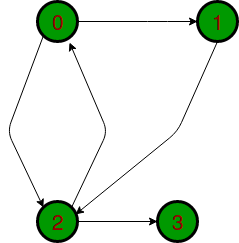Transitive closure of above graphs is
1 1 1 1
1 1 1 1
1 1 1 1
0 0 0 1
The graph is given in the form of adjacency matrix say ‘graph[V][V]’ where graph[i][j] is 1 if there is an edge from vertex i to vertex j or i is equal to j, otherwise graph[i][j] is 0.
Floyd Warshall Algorithm can be used, we can calculate the distance matrix dist[V][V] using Floyd Warshall, if dist[i][j] is infinite, then j is not reachable from I. Otherwise, j is reachable and the value of dist[i][j] will be less than V.
Instead of directly using Floyd Warshall, we can optimize it in terms of space and time, for this particular problem. Following are the optimizations:

1. Instead of an integer resultant matrix (dist[V][V] in floyd warshall), we can create a boolean reach-ability matrix reach[V][V] (we save space). The value reach[i][j] will be 1 if j is reachable from i, otherwise 0.
2. Instead of using arithmetic operations, we can use logical operations. For arithmetic operation ‘+’, logical and ‘&&’ is used, and for a min, logical or ‘||’ is used. (We save time by a constant factor. Time complexity is the same though)
Below is the implementation of the above approach
• C++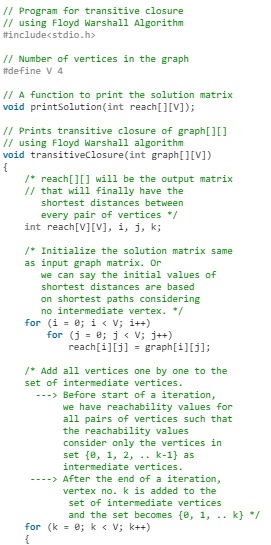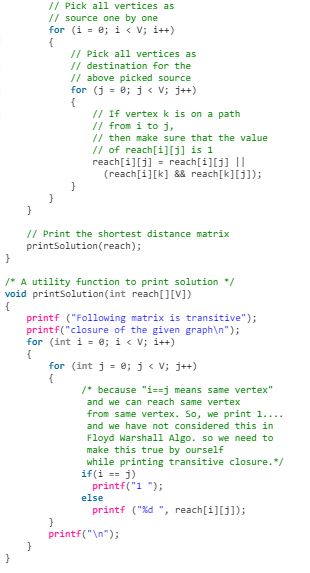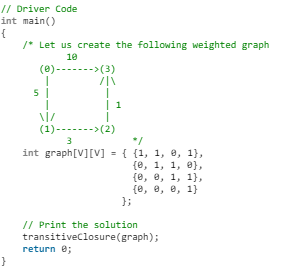• Java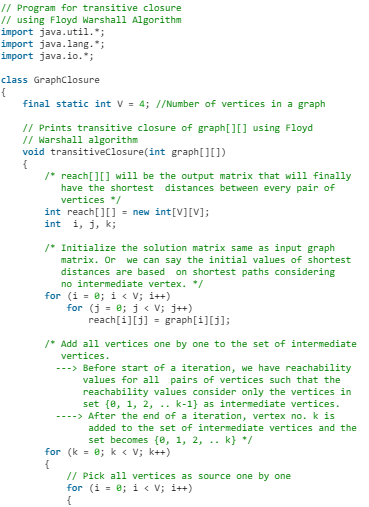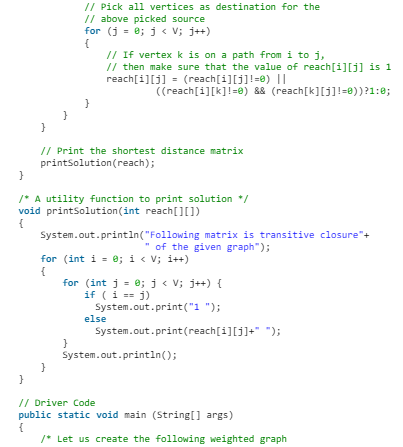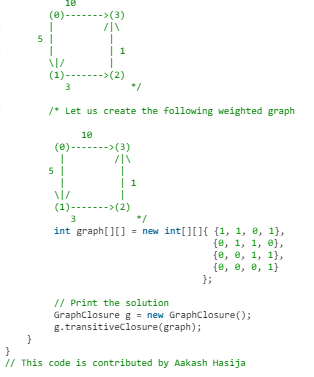• Python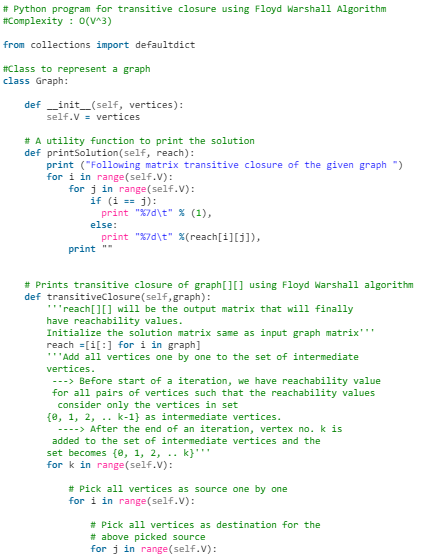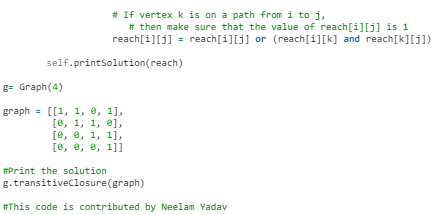• C#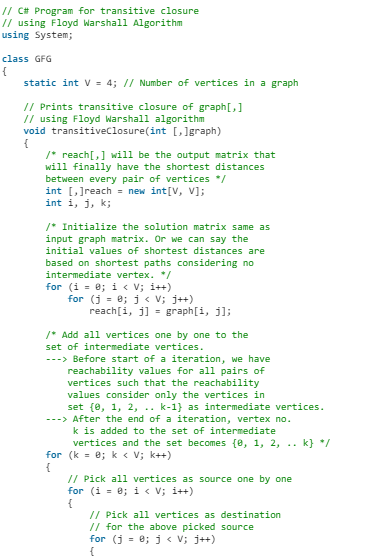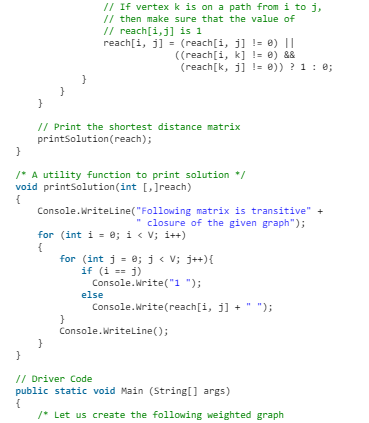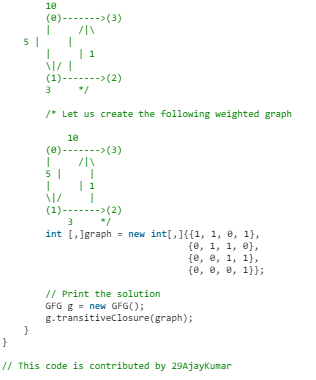Output
Following matrix is transitiveclosure of the given graph
1 1 1 1
0 1 1 1
0 0 1 1
0 0 0 1
Time Complexity: O(V3) where V is number of vertices in the given graph.
See below post for a O(V2) solution.

Offer running on EduRev: Apply code STAYHOME200 to get INR 200 off on our premium plan EduRev Infinity!

52 docs|31 tests

,

,

,

,

,

,

,

,

,

,

,

,

,

,

,

,

,

,

,

,

,

;